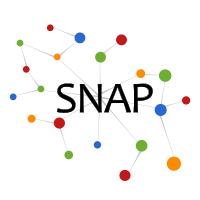Open positions
Open research positions in SNAP group are available here.

# Enhanced Hi-C interaction network

### Dataset information

This is a Hi-C interaction network. Hi-C is a chromosome conformation capture (3C)-based technology, an experimental approach for understanding chromatin interactions within DNA. The Hi-C technology measures pairwise chromatin interaction frequencies within a cell population. The genome is divided into bins of equal size (1K bases long), and the number of contacts observed between each pair of bins is reported. Nodes represent genomic regions and weighted edges represent normalized contacts between two regions in the genome.

Dataset statistics
Nodes (Chromosome 5) 7015
Edges (Chromosome 5) 434977
Nodes in largest SCC (Chromosome 5) 7015
Fraction of nodes in largest SCC (Chromosome 5) 1.000000
Edges in largest SCC (Chromosome 5) 434977
Fraction of edges in largest SCC (Chromosome 5) 1.000000
Average clustering coefficient (Chromosome 5) 0.633787
Number of triangles (Chromosome 5) 33808979
Fraction of closed triangles (Chromosome 5) 0.374705
Diameter (longest shortest path) (Chromosome 5) 4
90-percentile effective diameter (Chromosome 5) 2.974050
Nodes (Chromosome 15) 5746
Edges (Chromosome 15) 340118
Nodes in largest SCC (Chromosome 15) 5746
Fraction of nodes in largest SCC (Chromosome 15) 1.000000
Edges in largest SCC (Chromosome 15) 340118
Fraction of edges in largest SCC (Chromosome 15) 1.000000
Average clustering coefficient (Chromosome 15) 0.643585
Number of triangles (Chromosome 15) 25151820
Fraction of closed triangles (Chromosome 15) 0.353089
Diameter (longest shortest path) (Chromosome 15) 5
90-percentile effective diameter (Chromosome 15) 2.991200
Nodes (Chromosome 20) 3526
Edges (Chromosome 20) 231402
Nodes in largest SCC (Chromosome 20) 3526
Fraction of nodes in largest SCC (Chromosome 20) 1.000000
Edges in largest SCC (Chromosome 20) 231402
Fraction of edges in largest SCC (Chromosome 20) 1.000000
Average clustering coefficient (Chromosome 20) 0.770702
Number of triangles (Chromosome 20) 16149512
Fraction of closed triangles (Chromosome 20) 0.489030
Diameter (longest shortest path) (Chromosome 20) 5
90-percentile effective diameter (Chromosome 20) 3.469386

The network is constructed based on the raw contact map, which is normalized and denoised using network enhancement algorithm. Shown are network statistics for three human chromosomes, chromosome 5, 15, and 20.

### Files

File Size Description
GrGr-NE1K.tar.gz 35MB Enhanced Hi-C interaction networks and network statistics (one weighted network per human chromosome)